# RFEM

Online manuals, introductory examples, tutorials, and other documentation.

# 6.10 Free Polygon Loads

General description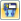A free polygon load acts as a uniform or linearly variable surface load on a polygonal, freely definable zone of a surface.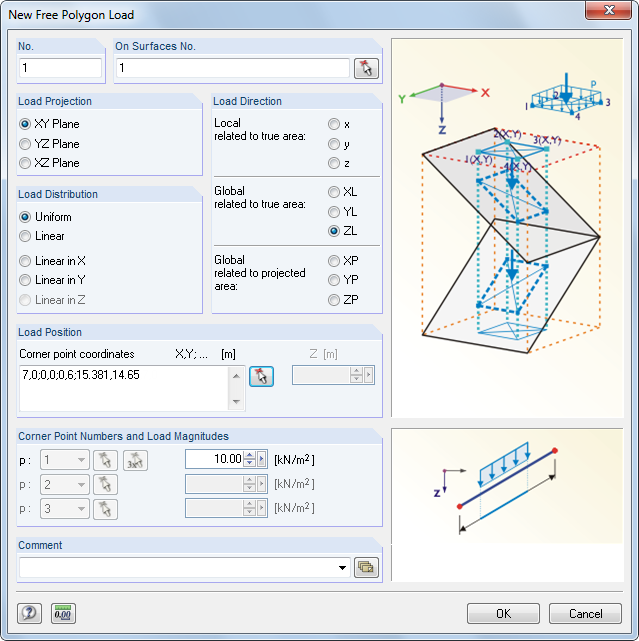Image 6.42 New Free Polygon Load dialog box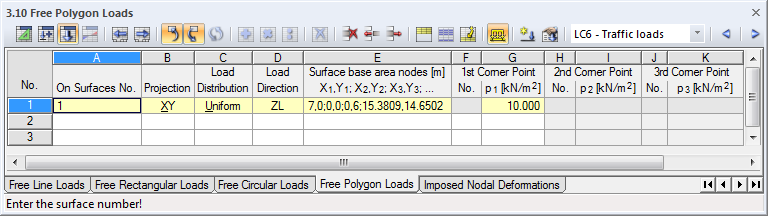Image 6.43 Table 3.10 Free Polygon Loads
On Surfaces No.

This text box manages the numbers of the surfaces on which the load acts. You can also select nodes graphically by using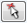.

Starting from the corner points defined by the Load Position, imaginary straight lines are "set" perpendicular to the projection plane. If these lines intersect any of the listed surfaces, the boundary line of the applied load is the connecting diagonal of both intersection points. In this way, it is possible to quickly allocate loads of a similar type to several surfaces.

Load Projection

The load can be projected onto one of the global planes XY, YZ, or XZ. As described above, imaginary straight lines are generated, starting from the load positions and running perpendicular to the projection plane. The corner points of the polygon load are assumed wherever the lines intersect a surface.

The projection plane must not be perpendicular to a surface on which the load acts: There are no clear points of intersection with the surface.

Load Distribution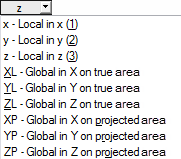Specify whether a uniform or linearly variable area load is applied. In the Corner Point Numbers and Load Magnitudes dialog section below, you can enter one (Uniform), two (Linear in X / Y / Z), or three (Linear) numerical values.

Load Direction

The load can act in direction of the global axes X, Y, Z or the local surface axes x, y, z. The load directions are described in Chapter 6.8.

Load Position

Enter the Corner point coordinates into the text boxes. It is recommended to graphically define the polygonal chain by using thebutton in the dialog box. In the text box or table column, enter the corner point coordinates separated by commas; coordinate pairs must be separated by a semicolon.

Example: corner point coordinates X, Y [m] 2, 3; 1.6, 4.7; 5, 45; 6, 25; 3, 2

The third coordinate component is automatically defined by the plane of load projection. In the example, it is the Z-coordinate that can be specified separately in a text box of the dialog box. When defining coordinates graphically, the component is irrelevant because the active work plane is decisive.

Corner Point Numbers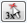When defining linearly variable area loads, enter two (Linear in X / Y / Z) or three (Linear) corner points with the corresponding load values. You can only define or graphically select corner points that are used to define the boundary lines in the Load Position dialog section. It is not possible to select RFEM nodes. Thus, the numbers of corner points are related to the sequence of the Corner point coordinates.

Load Magnitude

In this table column or text box, enter the numerical value of the area load. For a linearly variable distribution, two or three values must be entered.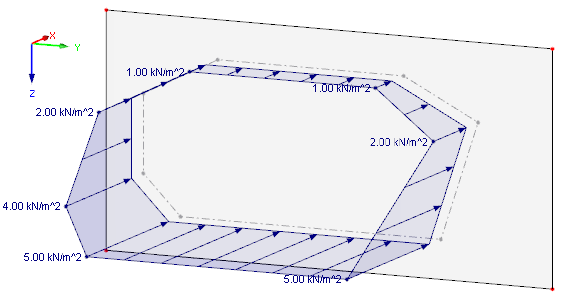Image 6.44 Polygon load that is linearly variable in direction Z: load projection plane YZ and local load direction in z